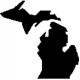# What Happens When the Interest Rate is Fixed?

Narayana Kocherlakota, president of the Minneapolis Fed made the following statement in a speech last week:

Long-run monetary neutrality is an uncontroversial, simple, but nonetheless profound proposition. In particular, it implies that if the FOMC maintains the fed funds rate at its current level of 0-25 basis points for too long, both anticipated and actual inflation have to become negative.

This set off a firestorm of criticism among a variety of folks in the blogosphere (just do a quick Google search). With that in mind, I thought that I would revisit an influential paper on the topic.

In 1992, Peter Howitt wrote a paper entitled, “Interest rate control and nonconvergence to rational expectations.” The purpose of the paper was to illustrate to many economists at the time the central insight of Wicksell’s cumulative process in which the control of interest rates by the central bank might lead to accelerating inflation (or deflation).

To illustrate this point, he used a model with the following two equations (an IS equation and a Phillips curve, respectively):

y(t) = -a[i(t) – pe(t) – r*]

p(t) = pe(t) + by(t)

where y(t) is output, i(t) is the nominal interest rate, pe(t) is expected inflation, r* is the natural real rate of interest, p(t) is inflation, and a and b are parameters.

Now suppose that the central bank decides to leave the interest rate fixed at ‘i’. Under rational expectations, in which p(t) = pe(t), there is one unique solution:

p(t) = p(t)* = i – r

where p(t)* is used to denote the rational expectations equilibrium rate of inflation. Thus, if the nominal interest rate is fixed at zero, the rate of inflation that prevails is

p(t) = -r

This seems to be where Kocherlakota is getting his conclusion. According to the model, under rational expectations the equilibrium inflation rate will be negative.

Now suppose that rational expectations does not hold. What will happen to inflation? Typically, it is assumed that even in the absence of rational expectations, individuals are able to learn from their forecast errors and therefore they ultimately end up at the ratex solution. Thus, it is important to consider what would happen in this model if forecast errors exist.

To obtain the possible forecast error, we can replace i(t) – r* in the IS equation with p(t)*, the rational expectations equilibrium solution. Now combine the IS equation and the Phillips curve by substituting for y in the Phillips curve. Doing so allows us to express the forecast error as follows:

p(t) – pe(t) = ab[pe(t) – p(t)*] (1)

Now suppose that the central bank sets the nominal interest rate such that the real rate of interest is lower than the natural rate of interest. Wicksell argued that in this scenario accelerating inflation would result. How can we test this in our model. Well, if the real rate of interest is less than the natural rate it must be true that:

r < r*

or

i – pe(t) < i – p(t)*

and thus

pe(t) > p(t)*

In other words, if the nominal rate of interest is set by the central bank such that the real rate of interest is lower than the natural rate, inflation expectations will be greater than the rational expectations equilibrium rate of inflation. Returning to equation (1), if inflation expectations are higher than the rational expectations equilibrium rate of inflation, the actual rate of inflation will be higher than the expected rate of inflation. If we assume that individuals have adaptive expectations, this means that they will revise their expectation of inflation upward. The importance of this finding is that even if individuals are able to learn from their forecast error, they will be led AWAY from the rational expectations equilibrium. In other words, it will not follow that expectations will eventually converge on the rational expectation.

So why is any of this important? Isn’t Kocherlakota simply claiming that we live in a world of rational expectations, which most macroeconomists endorse use?

It is important because Kocherlakota subsequently makes this statement:

If the FOMC hews too closely to conventional thinking, it might be inclined to keep its target rate low. That kind of reaction would simply re-enforce the deflationary expectations and lead to many years of deflation.

This statement seems to imply that the Fed should start thinking about raising interest rates. However, unless individuals have rational expectations from the outset (they perfectly forecast inflation), raising the interest rate is likely to push the real rate of interest (further) above the natural rate thereby causing (accelerating) deflation.

[A quick note: Howitt’s finding is robust to different learning mechanisms and to a flexible price framework. Interestingly enough, Howitt actually identifies what has come to be known as the Taylor principle in this paper as well.]

### 2 responses to “What Happens When the Interest Rate is Fixed?”

1.Jason Gillman

Which Came first.. The Chicken or the Egg?

Which is in the driver’s seat.. Inflation or interest rates?

Whomever is holding the interest rate fixed at an artificially low level will surely run out of money quickly, and must then make new money to continue. I don’t even want to imagine how much has been “fabricated”

You economist types are far too smart for this bumpkin.. All that formulaic stuff makes my eyeballs sweat. ;o)

2.Bryan

I’m not a macro person, but didn’t low central bank interest rates lead to deflation in Japan? Deflation, a housing bubble, and unproductive stimulus… why isn’t the U.S. learning from Japan’s mistakes?